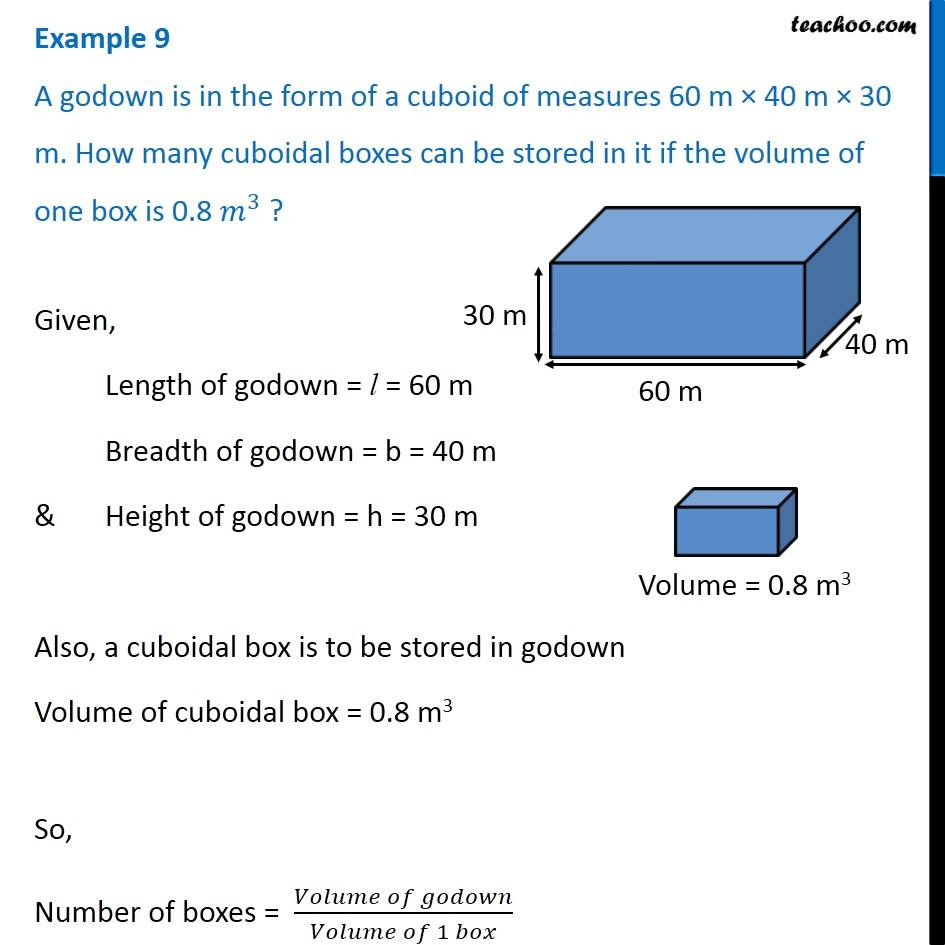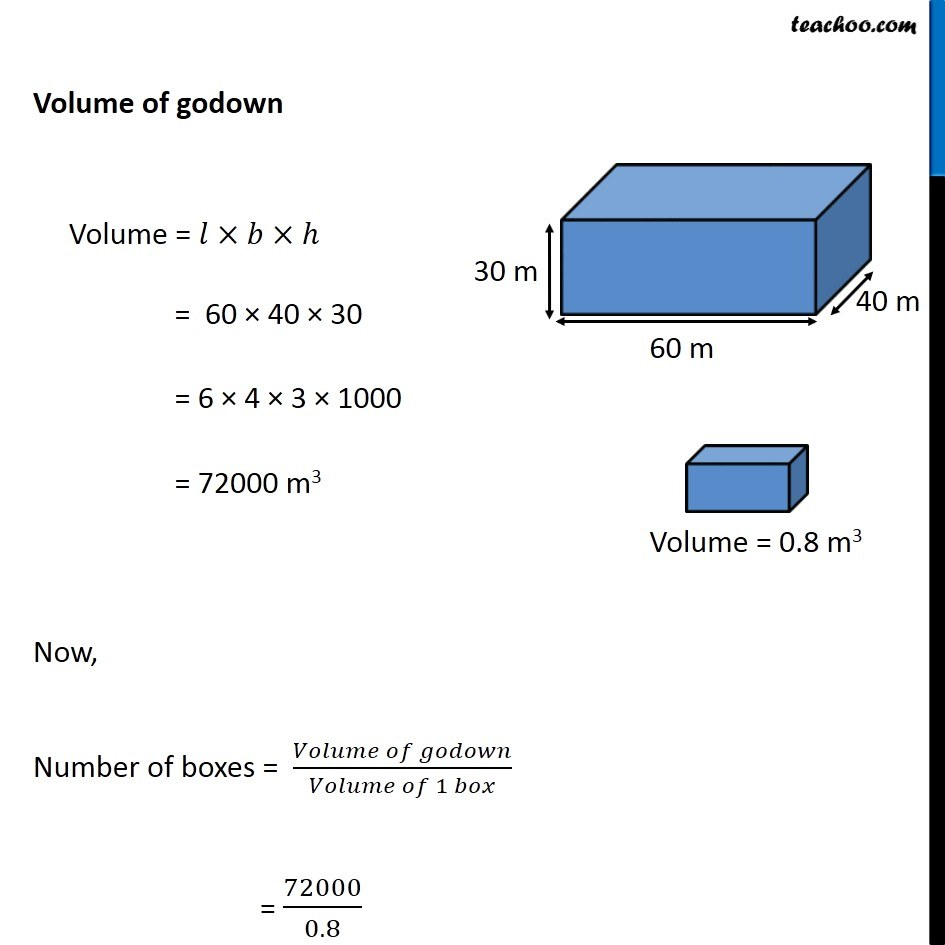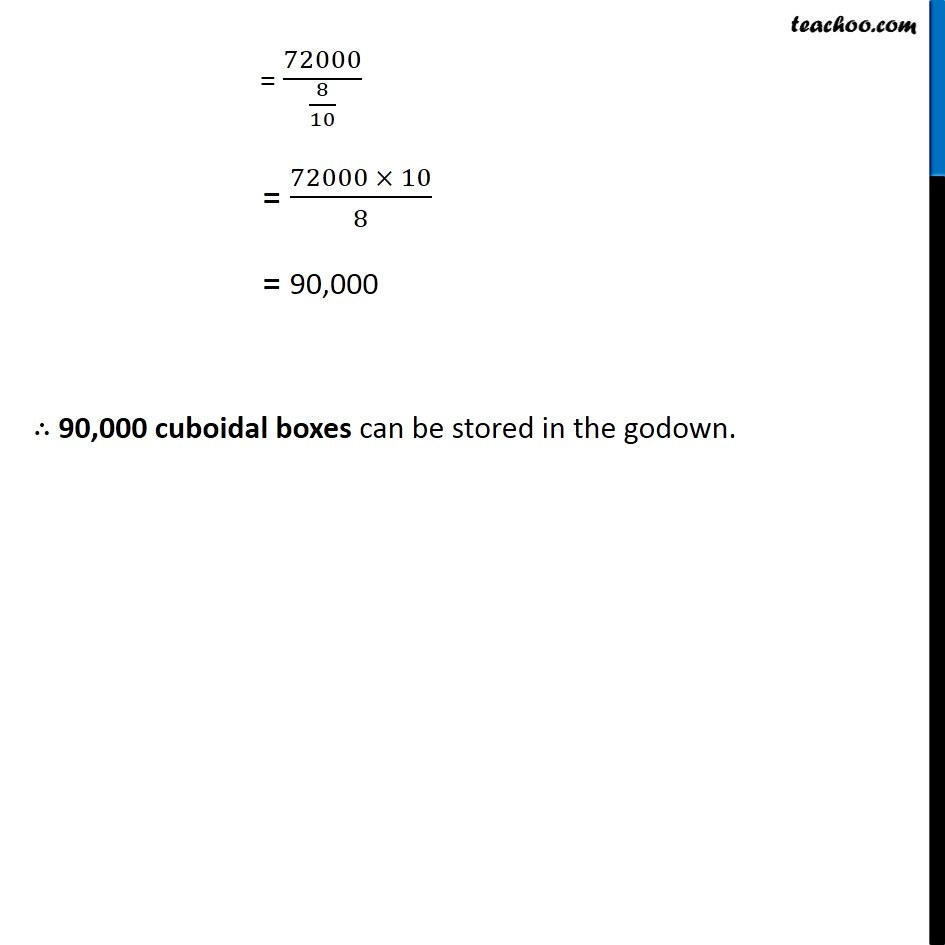Examples

Chapter 9 Class 8 Mensuration
Serial order wiseLearn in your speed, with individual attention - Teachoo Maths 1-on-1 Class

### Transcript

Example 9 A godown is in the form of a cuboid of measures 60 m × 40 m × 30 m. How many cuboidal boxes can be stored in it if the volume of one box is 0.8 𝑚^3 ? Given, Length of godown = l = 60 m Breadth of godown = b = 40 m & Height of godown = h = 30 m Also, a cuboidal box is to be stored in godown Volume of cuboidal box = 0.8 m3 So, Number of boxes = (𝑉𝑜𝑙𝑢𝑚𝑒 𝑜𝑓 𝑔𝑜𝑑𝑜𝑤𝑛)/(𝑉𝑜𝑙𝑢𝑚𝑒 𝑜𝑓 1 𝑏𝑜𝑥) Volume of godown Volume = 𝑙×𝑏×ℎ = 60 × 40 × 30 = 6 × 4 × 3 × 1000 = 72000 m3 Now, Number of boxes = (𝑉𝑜𝑙𝑢𝑚𝑒 𝑜𝑓 𝑔𝑜𝑑𝑜𝑤𝑛)/(𝑉𝑜𝑙𝑢𝑚𝑒 𝑜𝑓 1 𝑏𝑜𝑥) = 72000/0.8 = 72000/(8/10) = (72000 × 10)/8 = 90,000 ∴ 90,000 cuboidal boxes can be stored in the godown.